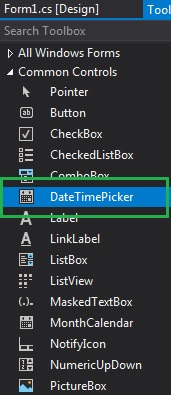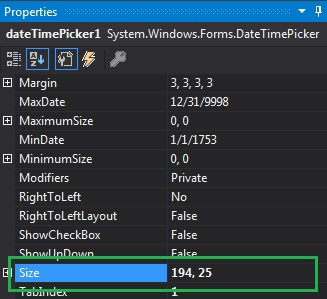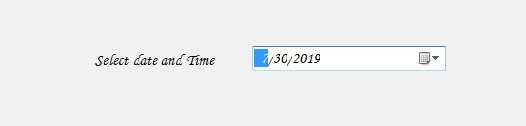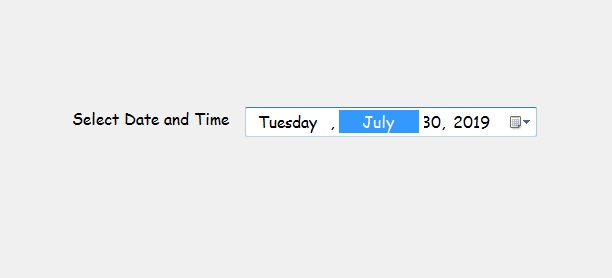# How to set the Size of the DateTimePicker in C#?

• Last Updated : 02 Aug, 2019

In Windows Forms, the DateTimePicker control is used to select and display date/time with a specific format in your form. In DateTimePicker control, you can set the size of the DateTimePicker using the Size Property. This property represents both height and width in pixels. You can set this property in two different ways:

1. Design-Time: It is the easiest way to set the Size of the DateTimePicker as shown in the following steps:

• Step 1: Create a windows form as shown in the below image:
Visual Studio -> File -> New -> Project -> WindowsFormApp• Step 2: Next, drag and drop the DateTimePicker control from the toolbox to the form as shown in the below image:• Step 3: After drag and drop you will go to the properties of the DateTimePicker and set the Size of the DateTimePicker as shown in the below image:Output:2. Run-Time: It is a little bit trickier than the above method. In this method, you can set the Size of the DateTimePicker control programmatically with the help of given syntax:

`public System.Drawing.Size Size { get; set; }`

Here, the Size indicates both height and width in pixels. The following steps show how to set the Size of the DateTimePicker dynamically:

• Step 1: Create a DateTimePicker using the DateTimePicker() constructor is provided by the DateTimePicker class.
```// Creating a DateTimePicker
DateTimePicker dt = new DateTimePicker();
```
• Step 2: After creating DateTimePicker, set the Size property of the DateTimePicker provided by the DateTimePicker class.
```// Setting the size
dt.Size = new Size(292, 26);
```
• Step 3: And last add this DateTimePicker control to the form using the following statement:
```// Adding this control to the form
```

Example:

 `using` `System;``using` `System.Collections.Generic;``using` `System.ComponentModel;``using` `System.Data;``using` `System.Drawing;``using` `System.Linq;``using` `System.Text;``using` `System.Threading.Tasks;``using` `System.Windows.Forms;`` ` `namespace` `WindowsFormsApp48 {`` ` `public` `partial` `class` `Form1 : Form {`` ` `    ``public` `Form1()``    ``{``        ``InitializeComponent();``    ``}`` ` `    ``private` `void` `Form1_Load(``object` `sender, EventArgs e)``    ``{``        ``// Creating and setting the``        ``// properties of the Label``        ``Label lab = ``new` `Label();``        ``lab.Location = ``new` `Point(183, 162);``        ``lab.Size = ``new` `Size(172, 20);``        ``lab.Text = ``"Select Date and Time"``;``        ``lab.Font = ``new` `Font(``"Comic Sans MS"``, 12);`` ` `        ``// Adding this control to the form``        ``this``.Controls.Add(lab);`` ` `        ``// Creating and setting the ``        ``// properties of the DateTimePicker``        ``DateTimePicker dt = ``new` `DateTimePicker();``        ``dt.Location = ``new` `Point(360, 162);``        ``dt.Size = ``new` `Size(292, 26);``        ``dt.MaxDate = ``new` `DateTime(2500, 12, 20);``        ``dt.MinDate = ``new` `DateTime(1753, 1, 1);``        ``dt.Format = DateTimePickerFormat.Long;``        ``dt.Name = ``"MyPicker"``;``        ``dt.Font = ``new` `Font(``"Comic Sans MS"``, 12);``        ``dt.Visible = ``true``;``        ``dt.Value = DateTime.Today;`` ` `        ``// Adding this control ``        ``// to the form``        ``this``.Controls.Add(dt);``    ``}``}``}`

Output:My Personal Notes arrow_drop_up